# Debt Sculpting with Curved DSCR Using Double Goal Seek Method

This page discusses modelling techniques of computing sculpted debt repayments in the situation with an amount of debt given by the debt to capital ratio and a minimum DSCR.  If three parameters are fixed: (1) the size of the debt; (2) the minimum DSCR; and, (3) the average debt life, then the optimal DSCR may not be constant over the tenure of the debt. This modelling problem of sculpting debt repayments to achieve an non-constant DSCR that is described on this page is one of the most difficult problems in project finance. I must thank my friend Hedieh for explaining this issue to me and helping me with the concepts.

When the average debt life, the minimum DSCR and the amount of debt are fixed, the period by period repayment cannot be easily calculated (as contrasted for example, in a sculpting case where the constant DSCR drives the debt level and a formula can be used for computing repayment). Specifically, if the DSCR changes over the tenure of the debt, the repayment pattern of the debt cannot be defined by a simple formula such as CFADS/DSCR. The only definite in the case with the size of debt given and a debt life constraint is that at some point over the debt tenure, the DSCR cannot fall below the minimum DSCR.  Three spreadsheets are used to explain the process of sculpting with fixed debt, a minimum DSCR and an average debt life constraint. The first two spreadsheets demonstrate attempting to construct sculpting with excel goal seek and solver techniques.  The third spreadsheet includes macros and functions to compute the sculpted debt repayment in the case where the DSCR gradually reaches the minimum debt DSCR.

The discussion below deals with financial modelling issues associated with sculpting in the case of fixed debt and average loan life constraints.  Other pages address theoretical issues and more technical discussion of how to develop user defined functions.

## Base Calculations with No Taxes Using Solver and Goal Seek

In computing the debt repayment that does not have a constant DSCR, the following calculations are necessary before an optimise function is used:

1. The LLCR should be computed from the net present value of CFADS divided by the amount of debt outstanding.
2. Some kind of interpolation function can be used to move from a high DSCR (that allows more dividends) down to the minimum DSCR.
3. The average loan life should be computed (using either the SUMPRODUCT function with repayments and debt period divided by the loan balance or the sum of the closing debt balance divided by the initial debt balance).
4. The debt balance should be computed from the net present value of debt service.
5. The initial DSCR can be computed from the LLCR computed in step 1 and a constant factor
6. The interpolation between the intial DSCR and the minimum DSCR as well as the constant LLCR factor can be used to derive the DSCR interpolation.

Some of the steps used to compute the interpolated DSCR are shown below. The first screen shot demonstrates how the LLCR can be used if the DSCR is constant. The LLCR is the PV of the CFADS of 1,028 divided by the constrained debt of 720. Since the LLCR is equal to the DSCR when the DSCR is constant throught the debt life, the LLCR can be used to produce a constant DSCR over the debt term with sculpting:

PV of DS = Debt

The debt service in row 10 is computed as the CFADS divided by the computed LLCR.  In this case, the minimum DSCR is not ever in place.  In the first screenshot, the LLCR is used as the basis for computing a debt service and produces a DSCR that is constant throughout the tenure of the debt.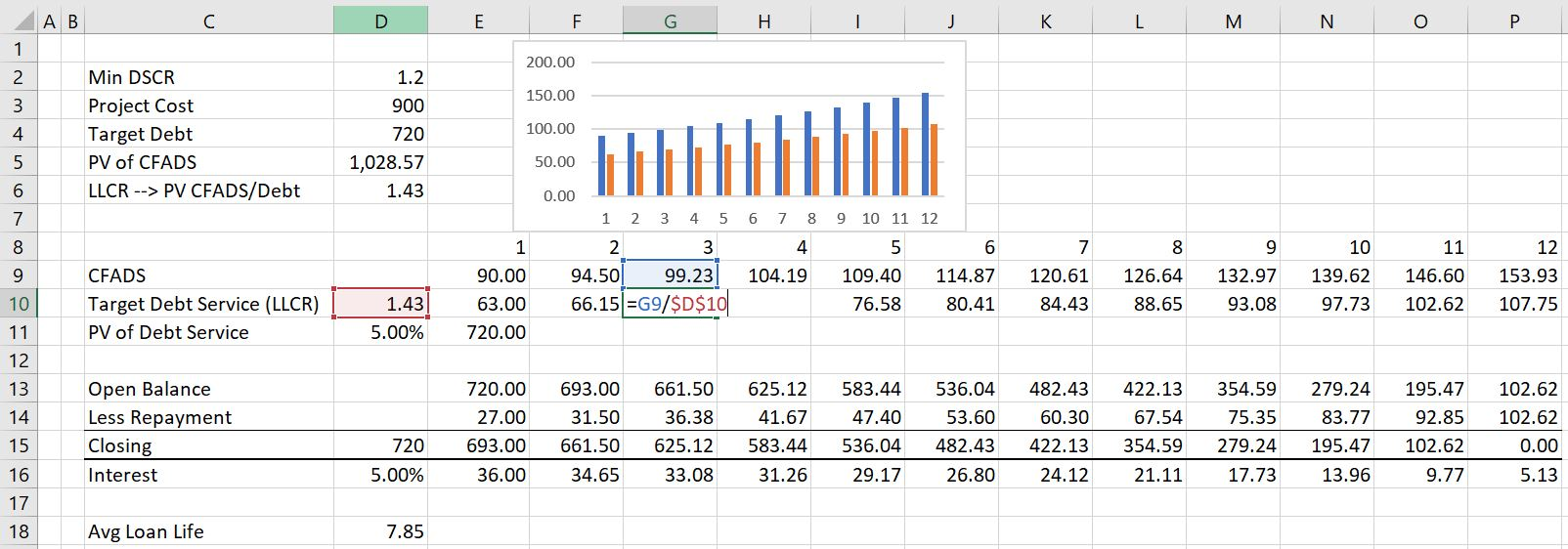In the second screenshot, the interpolate function (UDF) is used to move the DSCR from a high level down to the minimum level. In this case, the DSCR begins at 1.82 and by year 11 it falls to the minimum level of 1.2.  With these DSCR’s, the total debt is equal to the target debt of 700. The interpolate function is described in detail in the Excel Utilities section of the website. You can download the interpolate function file and copy the function to your project finance modelling file.The third screen shot shows calculation of the average loan life. There are two methods to compute the average loan life.  The first is using the SUMPRODUCT function and the second is using the sum of the closing balance divided the balance of the debt. The average debt life is necessary to compute because the average debt life is a constraint on the pattern of repayment.The next screen shot demonstrates how the interpolation factors can be computed.  The first factor is the final period before which the minimum DSCR is applied.  The second factor is the LLCR as computed above multiplied by an LLCR factor.  By changing both the final period and the LLCR factor, the progression of the DSCR over time can be changed. This change in the progression of the DSCR affects both the level of the debt and the average debt life.## Use of Double Goal Seek to Derive Sculpting Factors

In determining the final time period before the DSCR used to sculpt debt falls to the minimum DSCR and the LLCR factor that drives the initial DSCR, the two drivers drive two outputs.  These outputs are the level of the debt and the average debt life.  If the DSCR is too low, then the CFADS will be divided by a low number resulting in a high level of debt service and a high debt amount.  If the ending period before which the minimum DSCR is applied is too early then the average debt life will be very long. Through adjusting both the final period before the DSCR falls to the minimum and the initial level of the DSCR, a solution can potentially be found that results in both the target level of debt in the term sheet and the target average debt life in the term sheet.

In attempting to find the factors that optimise both the amount of the debt and the average debt life, you could try to use the goal seek tool or the solver tool in excel.  The first potential tool is the goal seek.  One could change the final period before which the DSCR falls to the minimum DSCR to adjust the level of debt.  This works when the LLCR factor is relatively high.  But when the LLCR factor is below 1.0, the goal seeks goes crazy (I think you know what I mean).  If the LLCR factor is above 1.0, the goal seek should work fine.  But if the LLCR is below 1.o, the goal seek does the crazy thing.  The problem is when you try and use the goal seek to optimise the average debt life. If the LLCR factor goes to below 1.0 after attempting a second debt life, the original goal seek for the debt size does not work.  This is illustrated in screenshot below.  In this screen shot, the debt balance should fall to zero and the end date should be above 2.0.  But because the LLCR factor is below 1.0, the goal seek does not work properly.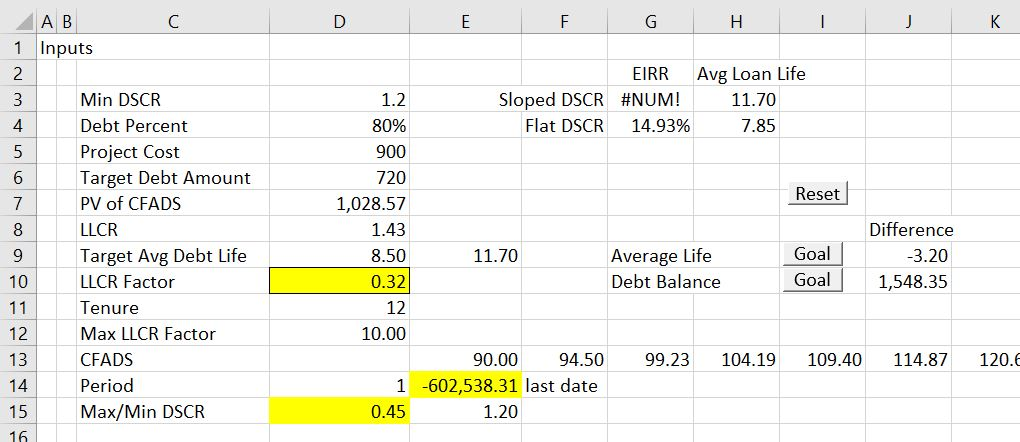The screenshot and the associated description above are meant to demonstrate that attempting the use two goal seeks can be very difficult in attempting to optimise the two parameters.  This is because after the second goal seek, the LLCR parameter can reach a level that causes the first goal seek to blow-up. If you run the goal seek that solves for the amount of debt and then manually change the LLCR factor, and then re-run the debt sizing goal seek, and then

## Solver Method to Optimise Two Variables with Two Inputs

Whenever you have multiple goal seeks that should work together, you can try use the solver tool in excel. Using constraints that set differences in target versus computed values to zero and making the changing cells the same as the goal seek changing cells, you can think of the solver tool as a multiple goal seek tool. In the context of the sculpting analysis this can sometimes work nicely but other times not work so well. If you do not have the solver installed, go to the options in excel and then select the Add-ins and find the solver by pressing “Go.”  The screenshot below illustrates how to set up the solver tool.  Note that the last date and the LLCR factor that have range names are in the changing cells section.  The constraints are that the difference between actual and computed debt is zero and the difference between the actual debt life and the target debt life is zero.While the solver tool can seem to be the ideal solution to the sculpting problem, there are a number of problems that include:

1. When alternative starting points are used, the solver does not work and a message that states a solution could not be found arises. For example if you enter the LLCR factor as 0.5, the solver fails to find a solution. A similar problem where the solver does not work occurs when the LLCR factor has a high value of something like 30.

2. If taxes are included in the analysis, there is an additional constraint driven by the circular reference from the interest deduction in computing taxable income. Specifically, the issue is related to computing the LLCR from PV of CFADS divided by target debt. To solve the problem, (1) the PV of CFADS can be fixed;  (2) the fixed amount of PV is compared to the computed amount; and, (3) a solver equation can be added to set the difference to zero by changing the fixed amount. When the circular reference constraint is added to the end time and the LLCR factor constraint, the solver function is less stable as shown on the second screenshot below.

3. To address circular references in a comprehensive manner it may be clumsy to use the solver.  For example, the tenure may change, DSRA income or DSRA L/C fees may have to be included and a host of other details can arise.  Many of these factors related to the DSRA can cause circular reference problems and require changing the set-up of the solver.  These issues may result in the solver failing.  For this reason, I have created a user defined function that replicates in a crude manner what the solver does.

4. In attempting to find a pattern of DSCR ratios that produce the target average life and the correct debt balance, there are situations where a solution is not possible. One example is when the target debt life is impossible to obtain with the tenure, minimum DSCR and the interest rate. A second example is when the average debt life is less than the debt life that would be produced by a simple LLCR technique. In both or these situations the solver will continue iterating and will not work. The problem is that you may not necessarily know why.

The screenshot below illustrates a case where the solver is not working.  In this example note the extreme initial DSCR which occurs after the solver has clunked along for a long time.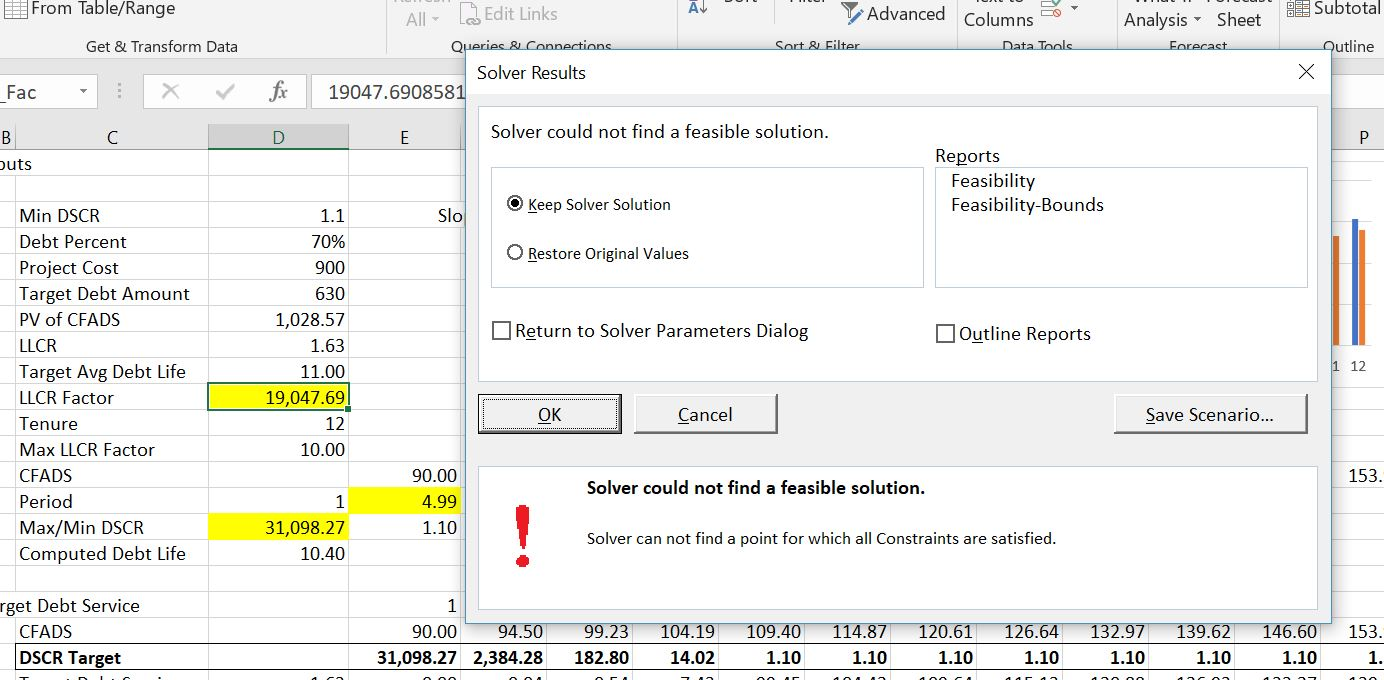The screenshot below demonstrates using the solver in a case with taxes.  I have used range names for the various factors.  In this case, by adding a separate equation for resolving the circular reference, the solver successfully resolves optimisation of the debt life, the debt amount and the circular reference associated with the present value of CFADS (CFADS is after tax).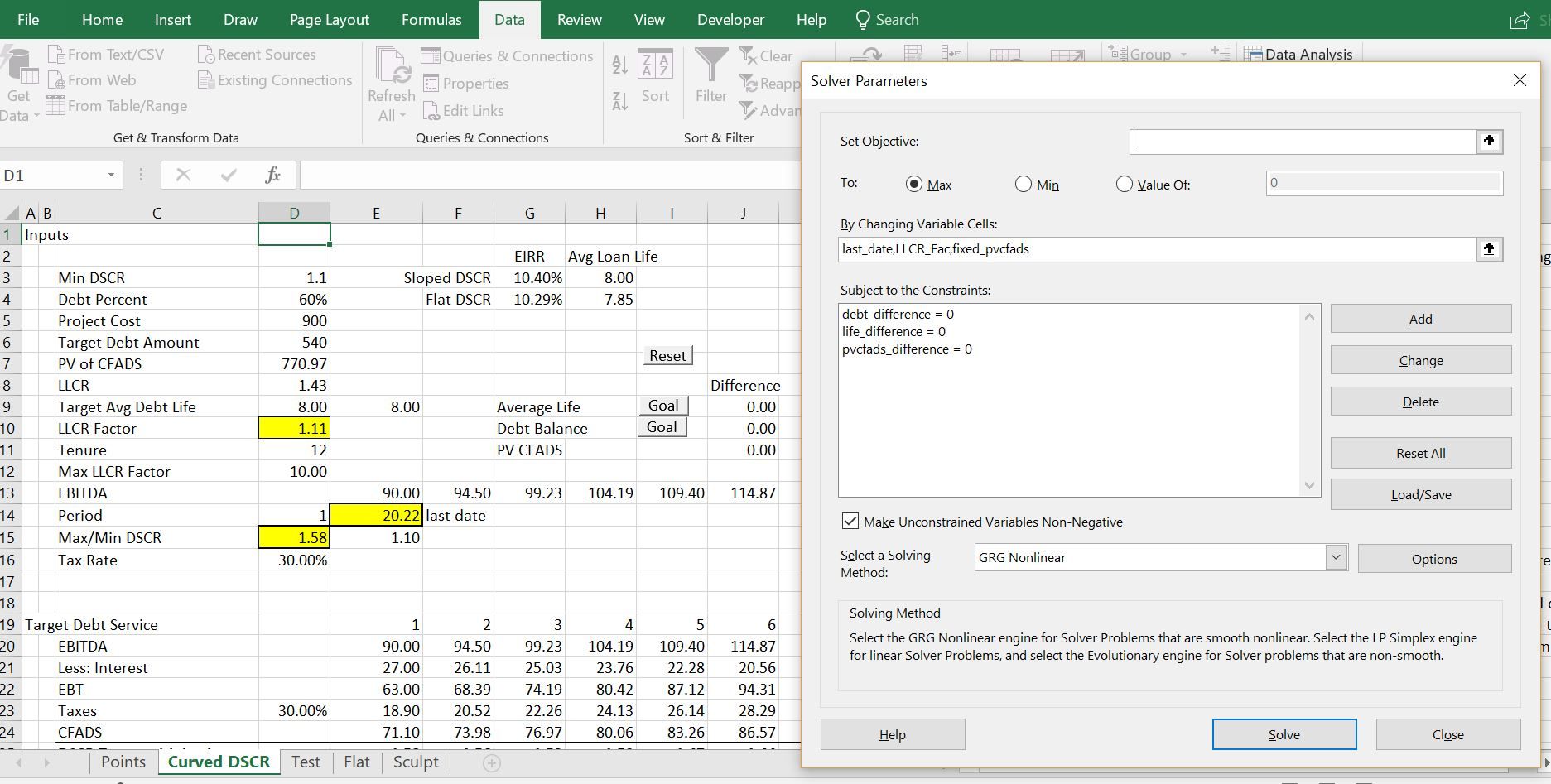## User-Defined Function to Optimise Debt Life and Debt Amount with Curved DSCR

Rather than use the solver or goal seeks, I have made a UDF to optimise the DSCR and debt repayment. In mimicking the solver, the iteration process is more tricky than creating a couple of dynamic goal seek functions.  To think about how the UDF works, you could use the simple example and press goal seek, then manually adjust the LLCR factor in the correct direction.  Then you could press the goal seek again and again adjust the LLCR factor. You could keep doing this until both the average debt life is equal to the target debt life and the debt balance equals the target debt balance driven by the debt to capital ratio.

If you are working on this sculpting problem, I recommend that you open the file and try to experiment with different parameters.  I should not say this because I think it is absurd to compare financial modelling with art, but when messing around with different cash flow patterns, minimum DSCR’s, target loan lives, debt to capital ratios etc., you can immediately see the effects on the DSCR, the repayment structure and the equity IRR.  I hope you try it even if you don’t understand all of the technical or even financial issues and understand what a financial model should really do.

A few illustrations of how the UDF works are demonstrated in screenshots below. The first screenshot demonstrates the general output of the UDF in a scenario where there are no problems with the iteration process. In this case there is a little note stating that the process has worked. You can change various parameters including the average debt life, the minimum DSCR and the debt size and evaluate the change in repayment patterns.A second case is demonstrated in the screenshot below where success is not obtained. You can think about things that will make the process not work.  One example is where the LLCR and the Minimum DSCR are close to one another.  In this case, trying to make the average debt life longer than the average life from flat case will not work. A second extreme example is when attempting to make the average debt life longer than is possible from the shape of the repayment curve.  There is a limit on the debt life relative to the debt tenure — if all the repayment occurs at the last year, then the average life will be equal to the tenure.  This situation where the tenure is equal to the average life of course is not possible because of the DSCR constraint, but the example does illustrate the constraint on too long a debt life.  The screenshot below demonstrates that when you make the target debt life too long, the iterative process cannot find a solution. But unlike the solver process, the lack of solution is clearly presented and you can quickly adjust different parameters to find better inputs.  The screenshot also shows that in extreme cases there is negative amortisation which will probably be unacceptable to the financial institution.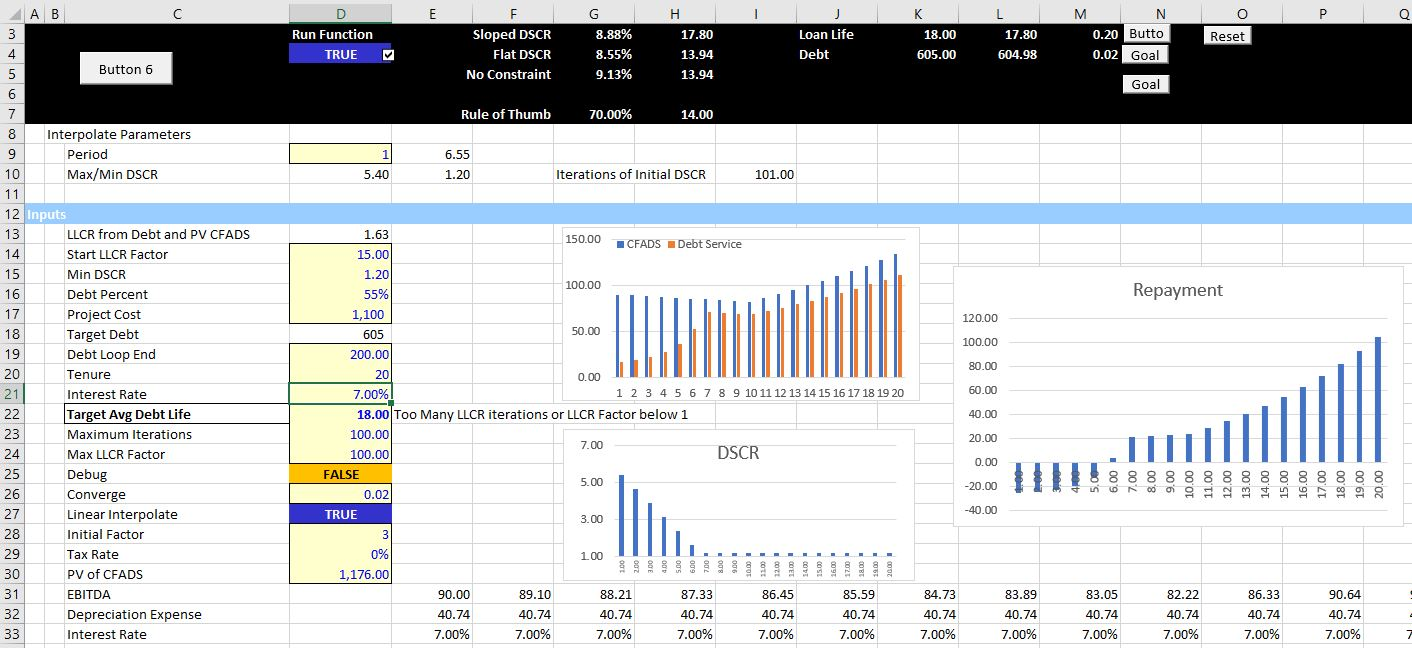## Changing the Function to a Macro to Verify the Iteration Routine

It has been painful to develop methods that will perform the iterations in an efficient manner and not result in a continual loop. You may want to inspect the iteration process yourself and see if you can find parameters to make the process more efficient. I hope this does not ever occur, but the function could result in an infinite loop. To allow you to inspect the iteration process, I have made the function so that you can convert it into a macro. When you convert the function to a macro, the various ways in which the goal seek loop for solving the debt balance and the LLCR factor iteration for solving the average life are documented.  The documentation is printed on a separate spreadsheet page.

If you would like to run the macro you have to change a couple of items in the code. You should remove the single inverted comma (‘) next to the function name.  You should also remove the single inverted comma from the Sub name. Finally you should change the function name variable from true to false in the code.  The code below illustrates how the items that you should change. The first excerpt shows the code in the function mode.  The second excerpt shows the code in the macro mode.

```.

'-------------------------------------------------------------------------------------------------------------------
' This is the function or macro sculpting routine
'-------------------------------------------------------------------------------------------------------------------

' Sub macro_optimise()

function_mode = True

.
.

'-------------------------------------------------------------------------------------------------------------------
' This is the function or macro sculpting routine
'-------------------------------------------------------------------------------------------------------------------

Sub macro_optimise()

' Function optimise(read_table, on_off_switch) As Variant

function_mode = False

.

```

To run the macro you can press the little cloud symbol at the top left of the spreadsheet as illustrated in screenshot below. If the inputs for debt life, minimum DSCR, debt tenor, debt size and EBITDA are the same (and the tax rate is zero), then the function and the macro should produce the same results. But if you run the macro, you can see details of how the process is converging and whether there are too many iterations or the process is bouncing back between variables.The screenshot below demonstrates outputs from the macro that document the iteration process.  The difference between the debt amount and the target debt amount as well as the difference between the debt life and the average debt life is shown on various lines.  When there are too many lines or when there is too much bouncing around the iteration function, you may have to go into the code and adjust the various factors.The sculpting methods discussed in other pages use what I have called the LLCR method to compute debt repayment with a constant DSCR when there is a debt to capital constraint.  For example, say the minimum DSCR is 1.35 and the maximum debt to capital ratio is 80%. Assume also that the debt to capital constraint of 80% is driving the size of the debt. In this case, you could compute the LLCR from the constrained debt and the PV of the CFADS.  If this LLCR is used, the DSCR will be constant, but higher than the minimum DSCR.  The LLCR method is reviewed in the screenshot below.

If the debt to capital constraint is limiting the debt size and the minimum DSCR is given in the term sheet, it is not necessary to keep the DSCR constant. Instead, you could increase the DSCR in some periods and then reduce the to reduce the debt repayment payments so as to achieve the debt to capital constraint. If the DSCR is allowed to fall to the minimum level over the life of the project, the DSCR does not have to be constant from period to period.  If the DSCR is allowed to be higher at the beginning of the debt life and then fall to the minimum level, then the debt repayments in the early part of the life of the project are reduced. This means that a level or flat DSCR over the debt life at a higher level than the LLCR computed flat amount is optimal. This idea is demonstrated in a simple example below with screenshots.

## Technical Discussion of VBA to Compute Curved DSCR

```.

For counter = 1 To 600

difference_debt = pv_debt_service - target_debt

'------------------------------------------------------------------------------------
' use lookup to get the dscr(i)
'------------------------------------------------------------------------------------

period_range(1) = start_time ' This changes each time
period_range(2) = end_time ' This changes each time

debt = target_debt

For i = 1 To tenure

If linear_interpolate = False Then dscr(i) = interpolate_look_up(i, period_range, dscr_range)
If linear_interpolate = True Then dscr(i) = interpolate_look_up_linear(i, period_range, dscr_range)

If tax_switch Then

interest = debt * interest_rate(i)
taxes(i) = (ebitda(i) - deb_exp(i) - interest) * tax_rate

End If

If dscr(i) <> 0 Then debt_service(i) = cfads(i) / dscr(i)

repayment = debt_service(i) - interest
debt = debt - repayment

Next i

pv_debt_service = WorksheetFunction.NPV(int_rate, debt_service)

' debt should get smaller as end time increases

debt_loop_count = debt_loop_count + 1
.

```